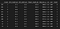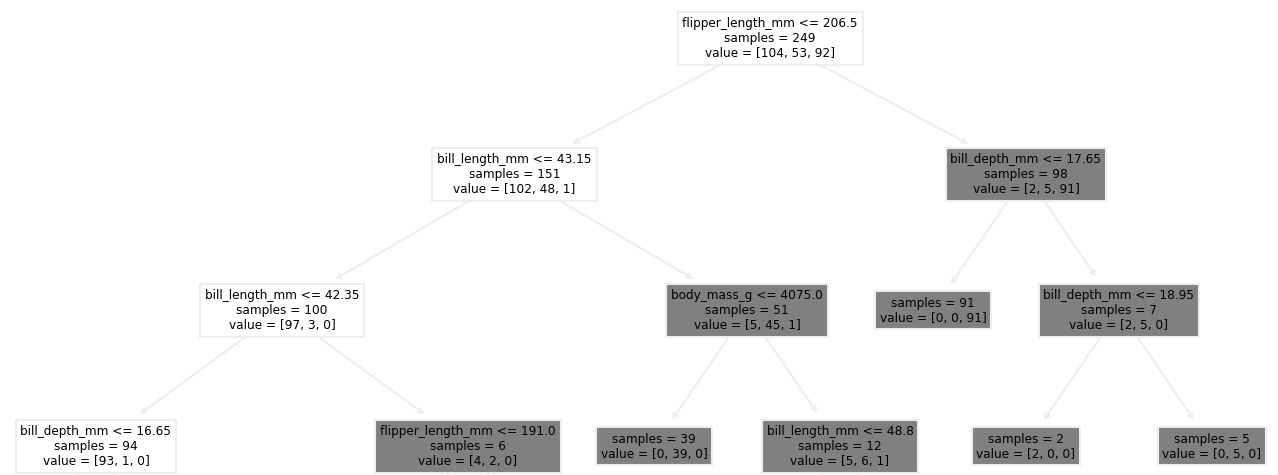# A (very) gentle introduction to multi-class classification

## Building a decision tree classifier with Python and Scikit-LearnPhoto by Ben Dove on Unsplash

Just like regression, classification algorithms are the bread to our data science butter and all serious data science enthusiasts should have a few classification algorithms under their belt. In this article, I will be taking you through the exploration of the penguins dataset and we will ultimately be fitting a decision tree classifier to predict a penguin’s species.

## The dataset

In this example, we will be using Python’s pmlb library and the penguins dataset. If you don’t have the pmlb library installed, go ahead and do so.

`pip install pmlb`

Next, we import our plotting libraries that will aid in the exploration and visualisation of the data.

`import seaborn as snsimport matplotlib.pyplot as pltplt.style.use('ggplot')`

We then fetch the penguins dataset.

`penguins = pmlb.fetch_data(“penguins”)`

Now that we have our data, the first step is to inspect the data that we are working with by taking a sample from the dataset.

`df.sample(10)`A random sample from the penguins dataset

We see that we have some measurements for the different penguin species — bill length and depth, flipper length, body mass — as well as the island where the species resides, the gender, year that the observation was made and the penguin’s species. The island, sex and target variables have already been encoded for us. (This article will point you in the right direction if you need some assistance on encoding categorical variables — I am a big fan of Pandas’ get_dummies).

## EDA (Exploratory Data Analysis)

Let’s start exploring the dataset and see if we can identify any specific relationships in the data.

My personal favourite way of getting to know the relationships between the various variables is to plot violin plots for each combination. A violin plot is essentially a box plot, but with the distribution of the dataset mirrored across the y-axis. In this case, it will help us determine what features could be used to differentiate the penguin species. We will be plotting the various penguin measurements against the target variable.

Although there are some obvious differences between the features for the different species, there is also significant overlap, especially for species 0 and 1. Let’s keep digging.

Another simple way of inspecting the relationship between all combinations of variables is to generate a pairplot using the Seaborn library.

`sns.pairplot(data=df, hue='target', palette=sns.color_palette(palette='hls' ,n_colors=3))`

Again, we see significant overlap of features for the various species. Interestingly, though, we see that penguin species 0 only occurs on island 2.

Another great method of inspecting the relationships between the variables is to plot a heatmap of the correlation. Correlation shows the strength of the linear relationship between two variables (as one variable increases/decreases, by how much does the other variable increase/decrease). A value of 1 indicates perfect correlation, while 0 indicates no correlation.

`# Create the correlations dataframecorr = df.corr()#Plot the heatmap using the correlations dataframesns.heatmap(data=corr, annot=True)plt.title('Correlation heatmap')`

The heatmap shows us that flipper length is highly correlated with the target variable (0.85) , followed by body mass (0.75). It also tells us that sex and year have very little correlation.

## Creating the model

Now that we have a good understanding of the data that we are working with, we are ready to create our model. We will be fitting a decision tree classifier to the data.

The first step is to import the necessary libraries

`import pandas as pdfrom sklearn.tree import DecisionTreeClassifier, plot_treefrom sklearn.model_selection import train_test_split, cross_val_scorefrom sklearn.metrics import accuracy_score`

Split the dataframe into its features.

`X = df.iloc[:, :-1]`

Isolate the target variable.

`y = df.iloc[:, -1]`

Next, split the dataset into training and testing sets and fit the model.

`# Split data into training and test setX_train, X_test, y_train, y_test = train_test_split(X, y, random_state=0)# Fit data to tree-based classification modelclassifier = DecisionTreeClassifier(random_state=0)classifier.fit(X_train, y_train)# View the accuracy of the modely_pred = classifier.predict(X_test)test_score = accuracy_score(y_test, y_pred)`

Now that the model has been created, let’s check the accuracy of our classifier against the test data.

`# Print the test scoreprint("Accuracy score of the tree = {:2.2%}".format(test_score))`

Accuracy score of the tree = 100.00% — our model is 100% accurate, which is to be expected. Decision trees tend to overfit to the data and our model is most likely overfitted. We would need to be prune our tree to improve its predictive accuracy on unseen data and to create a more parsimonious tree (Ockham’s razor comes to mind). We won’t be covering pruning in this article, but this will point you in the right direction.

We can check the output of our decision tree using plt_tree — This provides us with an interpretable output from our model.

`# Show the first few levels of the treeplt.figure(figsize=[6,3], dpi=300)plot_tree(classifier, max_depth=3,feature_names=X_train.columns, impurity=False)plt.show()`Penguins classifier output

The first split of a decision tree indicates the most important feature. The first node of our tree confirms what we saw in our heatmap — flipper length seems to be the most important feature when determining the penguin species. (Need some help interpreting the output of a decision tree? This article can help)

## Conclusion

Decision trees are very powerful algorithms and are a great starting point for any data science problem. Tree-based models provide an interpretable output that can easily be communicated to all stakeholders, require little pre-processing or feature selection and are not as effected by outliers and missing data as other algorithms. Next time you have a regression or classification problem, look no further.

An industrial engineer with a passion for data science.

## More from Renier Meyer

An industrial engineer with a passion for data science.